Test: FIR System Structures - 2

# Test: FIR System Structures - 2

Test Description

## 10 Questions MCQ Test Signals and Systems | Test: FIR System Structures - 2

Test: FIR System Structures - 2 for Electrical Engineering (EE) 2023 is part of Signals and Systems preparation. The Test: FIR System Structures - 2 questions and answers have been prepared according to the Electrical Engineering (EE) exam syllabus.The Test: FIR System Structures - 2 MCQs are made for Electrical Engineering (EE) 2023 Exam. Find important definitions, questions, notes, meanings, examples, exercises, MCQs and online tests for Test: FIR System Structures - 2 below.
Solutions of Test: FIR System Structures - 2 questions in English are available as part of our Signals and Systems for Electrical Engineering (EE) & Test: FIR System Structures - 2 solutions in Hindi for Signals and Systems course. Download more important topics, notes, lectures and mock test series for Electrical Engineering (EE) Exam by signing up for free. Attempt Test: FIR System Structures - 2 | 10 questions in 10 minutes | Mock test for Electrical Engineering (EE) preparation | Free important questions MCQ to study Signals and Systems for Electrical Engineering (EE) Exam | Download free PDF with solutions
 1 Crore+ students have signed up on EduRev. Have you?
Test: FIR System Structures - 2 - Question 1

### Which of the following is the application of lattice filter?

Detailed Solution for Test: FIR System Structures - 2 - Question 1

Explanation: Lattice filters are used extensively in digital signal processing and in the implementation of adaptive filters.

Test: FIR System Structures - 2 - Question 2

### If we consider a sequence of FIR filer with system function Hm(z)=Am(z), then what is the definition of the polynomial Am(z)?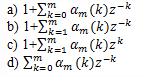Detailed Solution for Test: FIR System Structures - 2 - Question 2

Explanation: Consider a sequence of FIR filer with system function Hm(z)=Am(z), m=0,1,2…M-1
where, by definition, Am(z) is the polynomial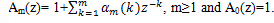Test: FIR System Structures - 2 - Question 3

### What is the unit sample response of the mth filter?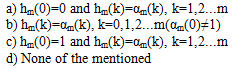Detailed Solution for Test: FIR System Structures - 2 - Question 3

Explanation: We know that Hm(z)=Am(z) and Am(z) is a polynomial whose equation is given as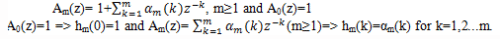Test: FIR System Structures - 2 - Question 4

The FIR filter whose direct form structure is as shown below is a prediction error filter.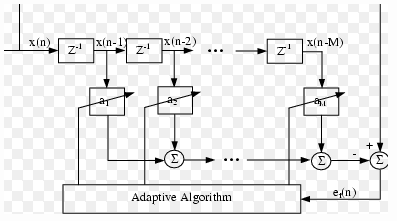Detailed Solution for Test: FIR System Structures - 2 - Question 4

Explanation: The FIR structure shown in the above figure is intimately related with the topic of linear prediction. Thus the top filter structure shown in the above figure is called a prediction error filter.

Test: FIR System Structures - 2 - Question 5

What is the output of the single stage lattice filter if x(n) is the input?

Detailed Solution for Test: FIR System Structures - 2 - Question 5

Explanation: The single stage lattice filter is as shown below.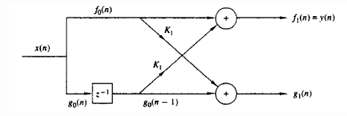Here both the inputs are excited and output is selected from the top branch.
Thus the output of the single stage lattice filter is given by y(n)= x(n)+Kx(n-1).

Test: FIR System Structures - 2 - Question 6

What is the output from the second stage lattice filter when two single stage lattice filers are cascaded with an input of x(n)?

Detailed Solution for Test: FIR System Structures - 2 - Question 6

Explanation: When two single stage lattice filters are cascaded, then the output from the first filter is given by the equation
f1(n)= x(n)+K1x(n-1)
g1(n)=K1x(n)+x(n-1)
The output from the second filter is obtained as
f2(n)=f1(n)+K2g1(n-1)
=x(n)+K2[K1x(n-1)+x(n-2)]+ K1x(n-1)
= x(n)+K1(1+K2)x(n-1)+K2x(n-2).

Test: FIR System Structures - 2 - Question 7

What is the value of the coefficient α2(1) in the case of FIR filter represented in direct form structure with m=2 in terms of K1 and K2

Detailed Solution for Test: FIR System Structures - 2 - Question 7

Explanation: The equation for the output of an FIR filter represented in the direct form structure is given as
y(n)=x(n)+ α2(1)x(n-1)+ α2(2)x(n-2)
The output from the double stage lattice structure is given by the equation,
f2(n)= x(n)+K2(1+K2)x(n-1)+K2x(n-2)
By comparing the coefficients of both the equations, we get
α2(1)= K1(1+K2).

Test: FIR System Structures - 2 - Question 8

The constants K1 and K2 of the lattice structure are called as reflection coefficients.

Detailed Solution for Test: FIR System Structures - 2 - Question 8

Explanation: The equation of the output from the second stage lattice filter is given by
f2(n)= x(n)+K1(1+K2)x(n-1)+K2x(n-2)
In the above equation, the constants K1 and K2 are called as reflection coefficients.

Test: FIR System Structures - 2 - Question 9

If a three stage lattice filter with coefficients K1=1/4, K2=1/2 K3=1/3, then what are the FIR filter coefficients for the direct form structure?

Detailed Solution for Test: FIR System Structures - 2 - Question 9

Explanation: We get the output from the third stage lattice filter as
A3(z)=1+(13/24)z-1+(5/8)z-2+(1/3)z-3.
Thus the FIR filter coefficients for the direct form structure are (1,13/24,5/8,1/3).

Test: FIR System Structures - 2 - Question 10

What are the lattice coefficients corresponding to the FIR filter with system function H(z)= 1+(13/24)z-1+(5/8)z-2+(1/3)z-3

Detailed Solution for Test: FIR System Structures - 2 - Question 10

Explanation: Given the system function of the FIR filter is
H(z)= 1+(13/24)z-1+(5/8)z-2+(1/3)z-3
Thus the lattice coefficients corresponding to the given filter is (1/4,1/2,1/3).

## Signals and Systems

32 videos|76 docs|63 tests
 Use Code STAYHOME200 and get INR 200 additional OFF Use Coupon Code
Information about Test: FIR System Structures - 2 Page
In this test you can find the Exam questions for Test: FIR System Structures - 2 solved & explained in the simplest way possible. Besides giving Questions and answers for Test: FIR System Structures - 2, EduRev gives you an ample number of Online tests for practice

## Signals and Systems

32 videos|76 docs|63 tests